Ex 7.1

Chapter 7 Class 10 Coordinate Geometry
Serial order wise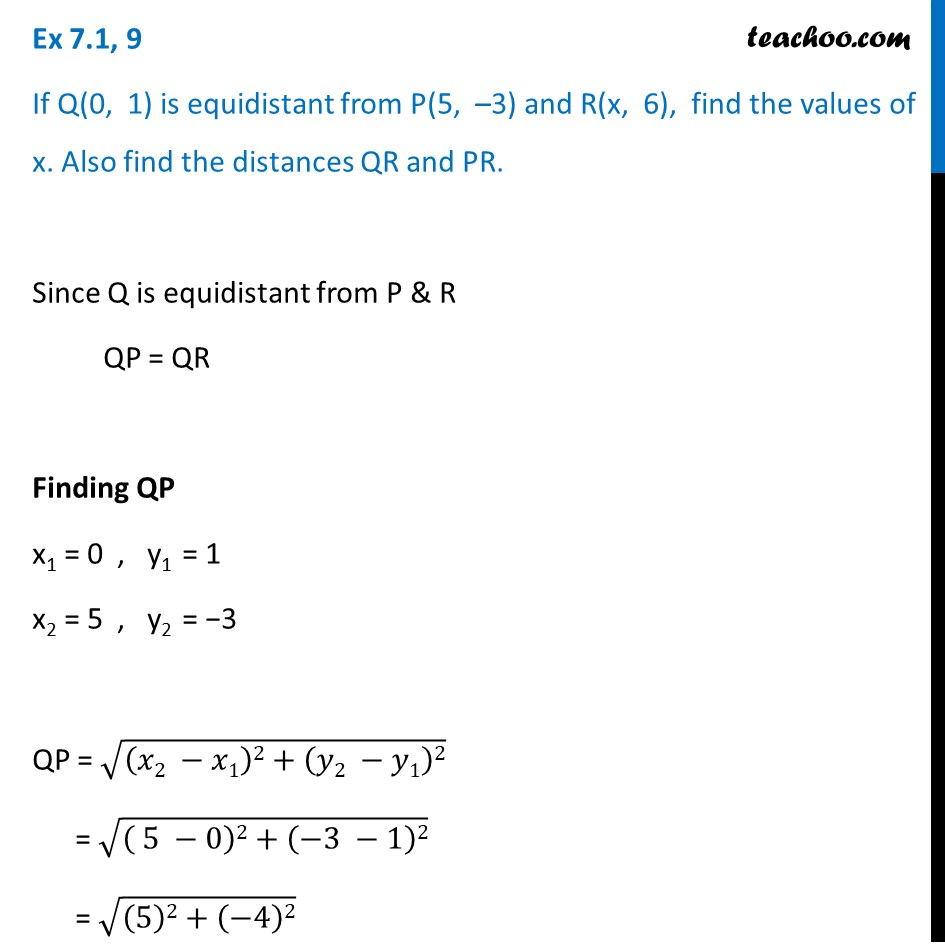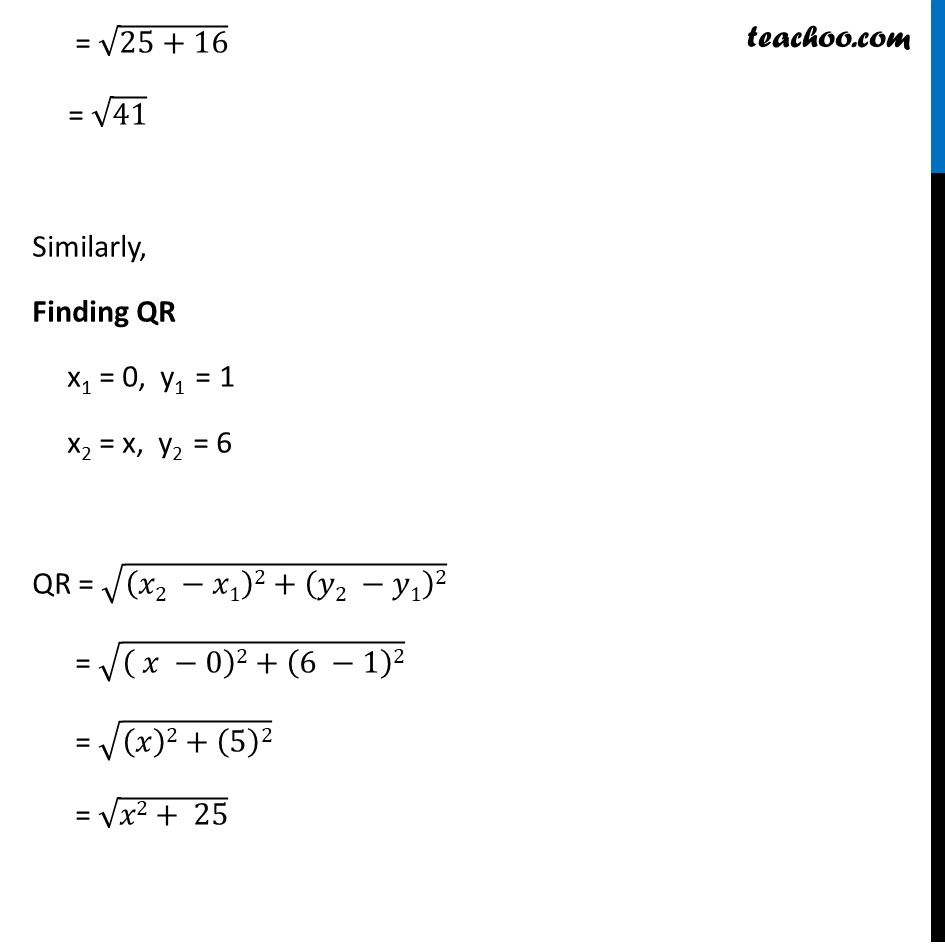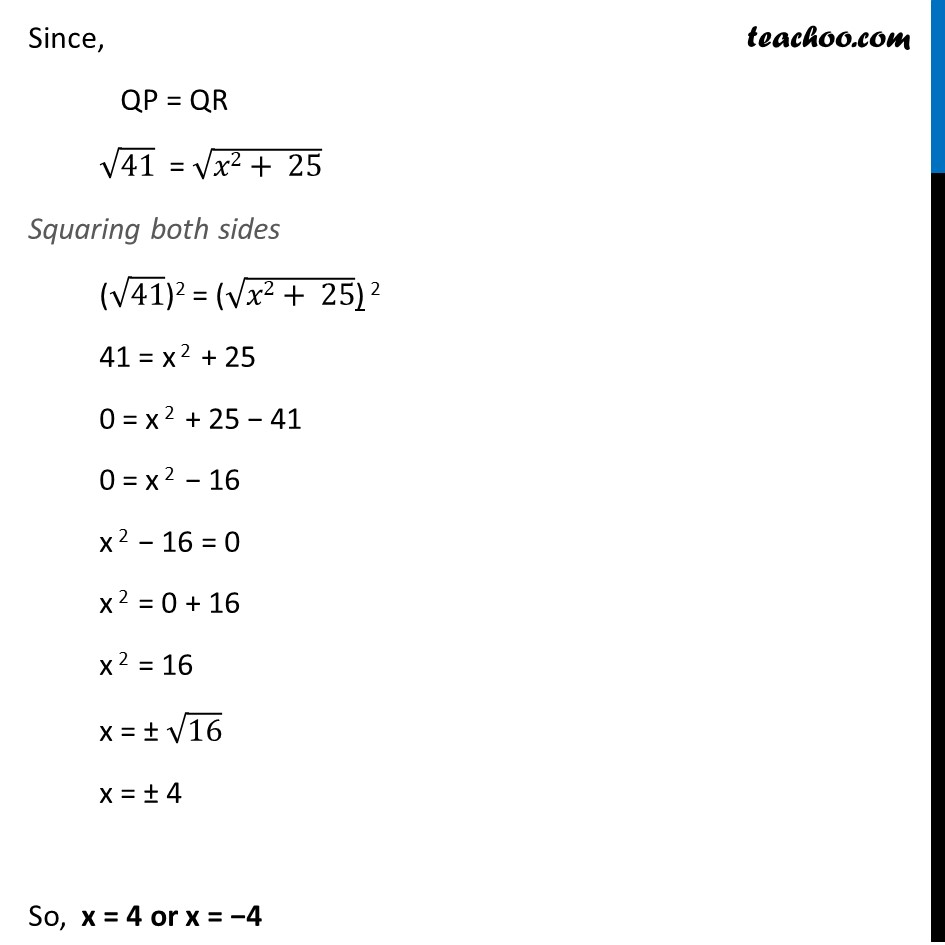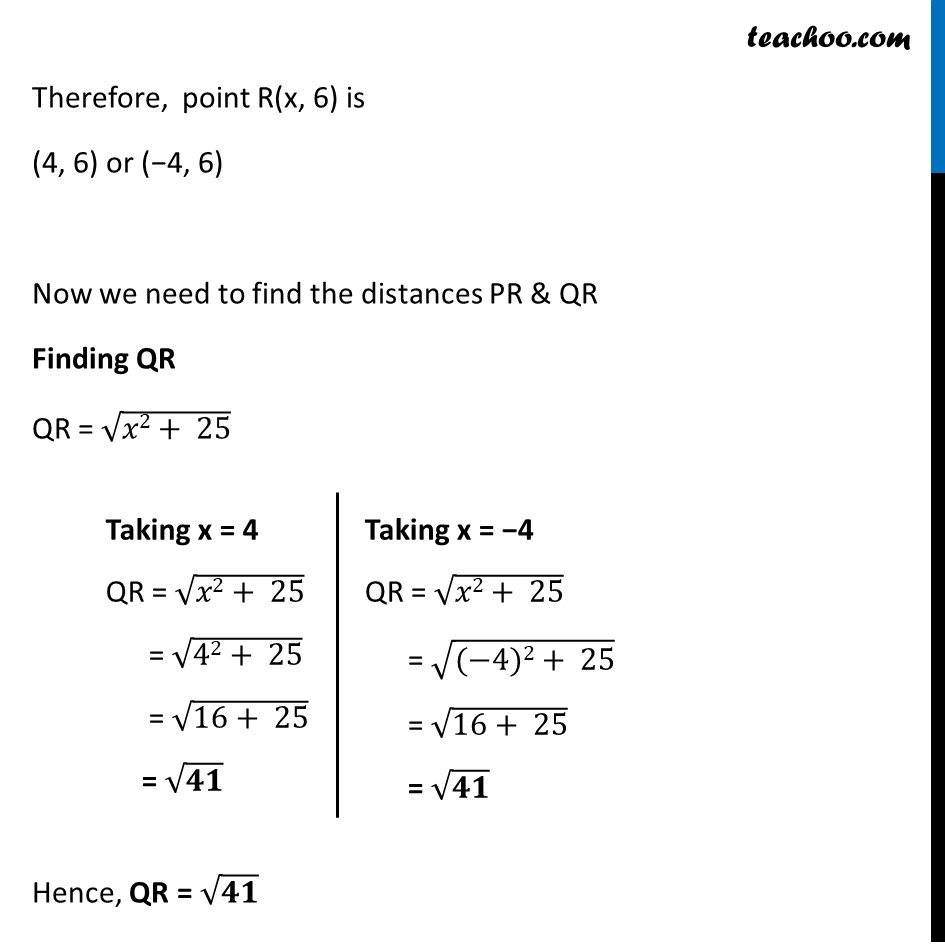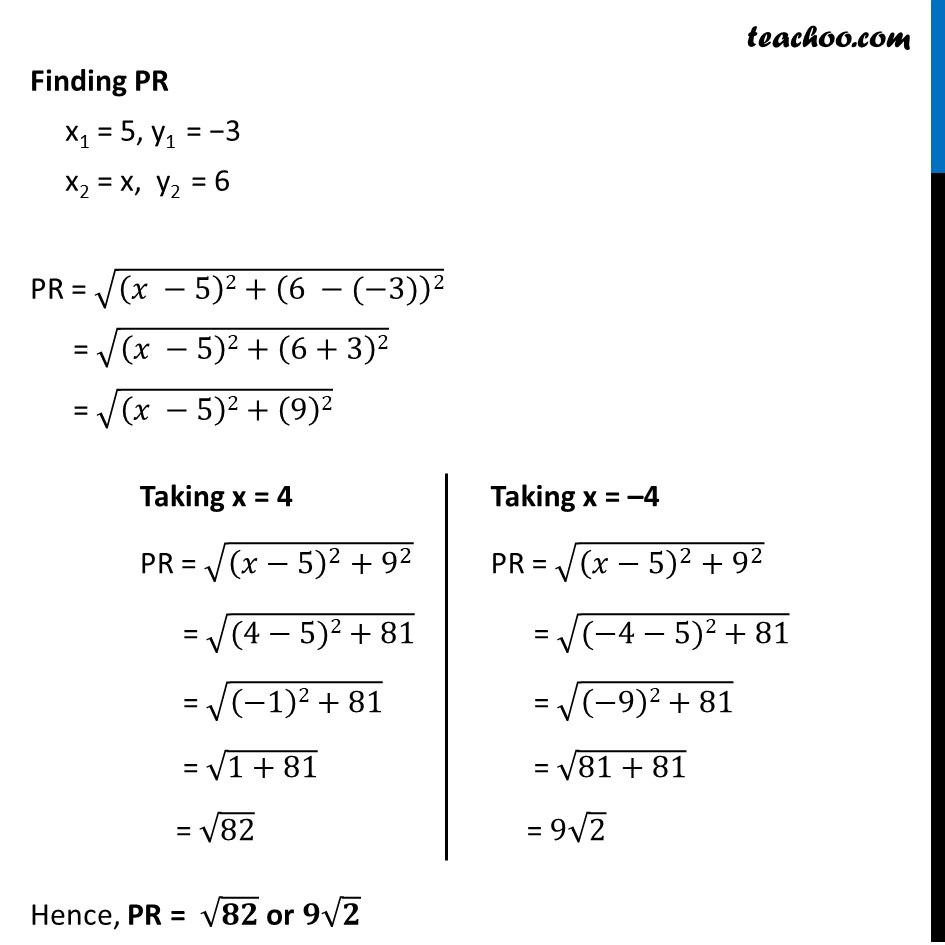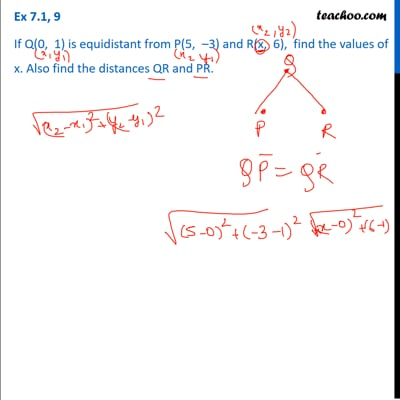This video is only available for Teachoo black users

### Transcript

Ex 7.1, 9 If Q(0, 1) is equidistant from P(5, –3) and R(x, 6), find the values of x. Also find the distances QR and PR. Since Q is equidistant from P & R QP = QR Finding QP x1 = 0 , y1 = 1 x2 = 5 , y2 = −3 QP = √((𝑥2 −𝑥1)2+(𝑦2 −𝑦1)2) = √(( 5 −0)2+(−3 −1)2) = √((5)2+(−4)2) = √(25+16) = √41 Similarly, Finding QR x1 = 0, y1 = 1 x2 = x, y2 = 6 QR = √((𝑥2 −𝑥1)2+(𝑦2 −𝑦1)2) = √(( 𝑥 −0)2+(6 −1)2) = √((𝑥)2+(5)2) = √(𝑥2+ 25) Since, QP = QR √41 = √(𝑥2+ 25) Squaring both sides (√41)2 = (√(𝑥2+ 25)) 2 41 = x 2 + 25 0 = x 2 + 25 − 41 0 = x 2 − 16 x 2 − 16 = 0 x 2 = 0 + 16 x 2 = 16 x = ± √16 x = ± 4 So, x = 4 or x = −4 Therefore, point R(x, 6) is (4, 6) or (−4, 6) Now we need to find the distances PR & QR Finding QR QR = √(𝑥2+ 25) Hence, QR = √𝟒𝟏 Taking x = 4 QR = √(𝑥2+ 25) = √(42+ 25) = √(16+ 25) = √𝟒𝟏 Taking x = −4 QR = √(𝑥2+ 25) = √((−4)2+ 25) = √(16+ 25) = √𝟒𝟏 Finding PR x1 = 5, y1 = −3 x2 = x, y2 = 6 PR = √((𝑥 −5)2+(6 −(−3))2) = √((𝑥 −5)2+(6+3)2) = √((𝑥 −5)2+(9)2) Hence, PR = √𝟖𝟐 or 𝟗√𝟐 Taking x = 4 PR = √((𝑥−5)^2+9^2 ) = √((4−5)2+81) = √((−1)2+81) = √(1+81) = √82 Taking x = –4 PR = √((𝑥−5)^2+9^2 ) = √((−4−5)2+81) = √((−9)2+81) = √(81+81) = 9√2